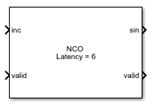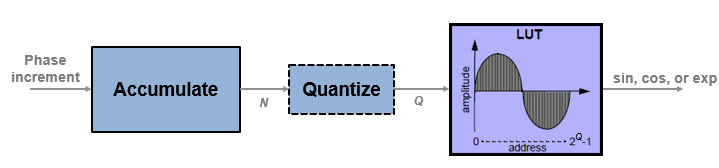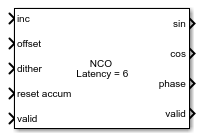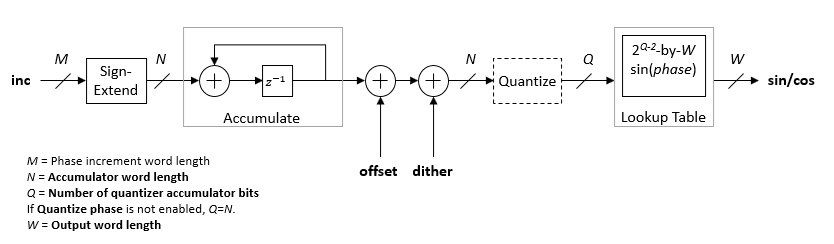# NCO

Generate real or complex sinusoidal signals

•Libraries:
DSP HDL Toolbox / Signal Operations
DSP HDL Toolbox / Sources

## Description

The NCO block generates real or complex sinusoidal signals, while providing hardware-friendly control signals.

A numerically-controlled oscillator (NCO) accumulates a phase increment and uses the quantized output of the accumulator as the index to a lookup table that contains the sine wave values. The wrap around of the fixed-point accumulator and quantizer data types provide periodicity of the sine wave, and quantization reduces the necessary size of the table for a given frequency resolution.For an example of how to generate a sine wave using the NCO block, see Generate Sine Wave. For more information on configuration and implementation, refer to the Algorithms section.

The block also provides these features:

• Optional frame-based output.

• A lookup table compression option to reduce the lookup table size. This compression results in less than one LSB loss in precision. See Lookup Table Compression for more information.

• An optional input port for external dither.

• An optional reset port that resets the phase accumulator to its initial value.

• An optional output port for the current NCO phase.

## Ports

Note

• This block appears in the Sources libraries with Phase increment source parameter set to `Property`. The only input port is valid.

• This block appears in the Signal Operations libraries with Phase increment source parameter set to `Input port`. This configuration shows the optional input port inc.

This icon shows the optional ports of the NCO block.### Input

expand all

Phase increment, specified as a scalar integer. The block casts this value to match the accumulator word length.

`double` and `single` data types are supported for simulation but not for HDL code generation.

#### Dependencies

To enable this port, set the Phase increment source parameter to ```Input port```.

Data Types: `single` | `double` | `int8` | `int16` | `int32` | `int64` | `uint8` | `uint16` | `uint32` | `uint64` | `fixdt([],N,0)`

Phase offset, specified as a scalar integer.

`double` and `single` data types are supported for simulation but not for HDL code generation.

#### Dependencies

To enable this port, set the Phase offset source parameter to ```Input port```.

Data Types: `single` | `double` | `int8` | `int16` | `int32` | `int64` | `uint8` | `uint16` | `uint32` | `uint64` | `fixdt([],N,0)`

Dither, specified as an integer or a column vector of integers. The length of the vector must equal the Samples per frame parameter value.

`double` and `single` data types are supported for simulation but not for HDL code generation.

#### Dependencies

To enable this port, set the Dither source parameter to `Input port`.

Data Types: `single` | `double` | `int8` | `int16` | `int32` | `int64` | `uint8` | `uint16` | `uint32` | `uint64` | `fixdt([],N,0)`

Control signal that enables NCO operation, specified as a Boolean scalar. When this signal is `1`, the block increments the phase and captures any input values. When this signal is `0`, the block holds the phase accumulator and ignores any input values.

When the Samples per frame parameter is greater than `1`, this value enables processing of Samples per frame samples.

Data Types: `Boolean`

Control signal that resets the accumulator, specified as a Boolean scalar. When this signal is `1`, the block resets the accumulator to its initial value. This signal does not reset the output samples in the pipeline.

#### Dependencies

To enable this port, select the Enable accumulator reset input port parameter.

Data Types: `Boolean`

### Output

expand all

Generated waveform, returned as a scalar or as a column vector with length equal to the Samples per frame parameter value. The output can be a single port that returns sin or cos values, a single port that returns exp values representing `cosine + j*sine`, or two ports that return sin and cos values, respectively.

When all input values are fixed-point type or all input ports are disabled, the block determines the output type using the Output data type parameter. When any input value is floating-point type, the block ignores the Output data type parameter. In this case, the block returns the waveform as floating-point values. Floating-point data types are supported for simulation but not for HDL code generation.

#### Dependencies

By default, this output port is a sine wave, sin. The port label and format changes based on the Type of output signal parameter.

Current phase of NCO, returned as a scalar or as a column vector with length equal to the Samples per frame parameter value. The phase is the output of the quantized accumulator with offset and increment applied. If quantization is disabled, this port returns the output of the accumulator with offset and increment applied. The values are of type `fixdt(1,N,0)`, where `N` is the Number of quantizer accumulator bits parameter value. If quantization is disabled, then `N` is the Accumulator Word length parameter value.

If any input value is floating-point type, the block returns the phase as a floating-point value. Floating-point data types are supported for simulation but not for HDL code generation.

#### Dependencies

To enable this port, select the Enable phase port parameter.

Data Types: `single` | `double` | `fixdt(1,N,0)`

Control signal that indicates validity of output data, returned as a Boolean scalar. When output valid is `1`, the values on the sin, cos, exp, and phase ports are valid. When output valid is `0`, the values on the output ports are not valid.

When the Samples per frame parameter is greater than `1`, this signal indicates the validity of all elements in the output vector.

Data Types: `Boolean`

## Parameters

expand all

### Main

Note

This block supports `double` and `single` input for simulation but not for HDL code generation. When all input values are fixed-point type or all input ports are disabled, the block determines the output type using the Output data type parameter. When any input value is floating-point type, the block ignores the Output data type parameter. In this case, the block returns the waveform and optional phase as floating-point values.

To use the Fixed-Point Designer™ data type override feature, you can obtain a `double` output value by applying `double` input data to one of the optional ports.

You can set the phase increment with an input port or by entering a value for the parameter. If you select `Property`, the Phase increment parameter appears for you to enter a value. If you select `Input port`, the inc port appears on the block.

Phase increment for the generated the waveform, specified as an integer. The block casts this value to match the accumulator word length.

#### Dependencies

To enable this parameter, set the Phase increment source parameter to `Property`.

Data Types: `single` | `double` | `int8` | `int16` | `int32` | `uint8` | `uint16` | `uint32` | `fixdt([],N,0)`

You can set the phase offset with an input port or by entering a value for the parameter. If you select `Property`, the Phase offset parameter appears for you to enter a value. If you select `Input port`, the offset port appears on the block.

Phase offset for the generated waveform, specified as an integer.

#### Dependencies

To enable this parameter, set the Phase offset source parameter to `Property`.

Data Types: `single` | `double` | `int8` | `int16` | `int32` | `uint8` | `uint16` | `uint32` | `fixdt([],N,0)`

You can set the dither from an input port or from a parameter. If you select `Property`, the Number of dither bits parameter appears. If you select `Input port`, a port appears on the block. If you select `None`, the block does not add dither.

Number of dither bits, specified as a positive integer.

#### Dependencies

To enable this parameter, set the Dither source parameter to `Property`.

When you set this value to `1`, the block has scalar input and output. When this value is greater than `1`, the dither port expects a column vector of length Samples per frame and the sin, cos, exp, and phase ports return column vectors of length Samples per frame.

By default, the block implements a noncompressed lookup table, and the output of this block matches the output of the NCO block. When you enable this option, the block implements a compressed lookup table. The Sunderland compression method reduces the size of the lookup table, losing less than one LSB of precision. The spurious free dynamic range (SFDR) is empirically 1–3 dB lower than the noncompressed case. The hardware savings of the compressed lookup table allow room to improve performance by increasing the word length of the accumulator and the number of quantize bits. For detail of the compression method, see Algorithms.

Select this parameter to enable the reset accum port. When reset accum is `1`, the block resets the accumulator to its initial value.

If you select `Sine` or `Cosine`, the block shows the applicable port, sin or cos. If you select `Complex exponential`, the output is of the form `cosine + j*sine` and the port is labeled exp. If you select ```Sine and cosine```, the block shows two ports, sin and cos.

When you set the Type of output signal parameter to `Complex exponential` or ```Sine and cosine```, the block implements a 1/8 sine wave lookup table for each of the sine and cosine parts of the waveform, and uses control logic to select and invert the values to generate both sine and cosine waveforms. This optimization means that dual output mode uses similar hardware resources compared to single output mode.

Select this parameter to return the current NCO phase on the phase port. The phase is the output of the quantized accumulator, with offset and increment applied. If quantization is disabled, this port returns the output of the accumulator, with offset and increment applied.

### Data Types

Rounding mode for fixed-point operations. Rounding Mode is a read-only parameter with value `Floor`.

Overflow mode for fixed-point operations. Overflow mode is a read-only parameter. Fixed-point numbers wrap around on overflow.

Accumulator data type description. This parameter is read-only, with value `Binary point scaling`. The block defines the fixed-point data type using the Accumulator Signed, Accumulator Word length, and Accumulator Fraction length parameters.

This parameter is read-only. All output is signed format.

Units are in bits. This value must include the sign bit.

If you clear the Quantize phase parameter, then Accumulator word length determines the LUT size. For HDL code generation, the LUT size must be between 2 and 217 entries. When you select Enable look up table compression method, this parameter must be an integer in the range [5,21]. When you clear Enable look up table compression method, this parameter must be an integer in the range [3,19]. For more information on how this parameter affects the LUT size, see the Algorithms section.

When you select the Quantize phase parameter, there is no limit to the Accumulator word length parameter value.

This parameter is read-only. The accumulator fraction length is zero bits.

The accumulator operates on integers. If the phase increment is `fixdt` type with a fractional part, the block returns an error.

When you select Quantize phase, the block quantizes the result of the phase accumulator to a fixed bit-width. The block uses this quantized value to select a waveform value from the lookup table. Quantizing the output of the phase accumulator enables you to reduce the lookup table size without lowering the frequency resolution. Select the size of the lookup table by using the Number of quantizer accumulator bits parameter.

When you clear Quantize phase, the block uses the full accumulator value as the address of the lookup table.

Number of quantizer accumulator bits, specified as an integer scalar less than the accumulator word length. For HDL code generation, this parameter value must result in a LUT size between 2 and 217 entries. When you select Enable look up table compression method, this parameter must be an integer in the range [5,21]. When you clear Enable look up table compression method, this parameter must be an integer in the range [3,19]. For more information on how this parameter affects the LUT size, see the Algorithms section.

#### Dependencies

To enable this parameter, select the Quantize phase parameter.

Specify the data type for the sin, cos, and exp ports. This parameter is ignored if any input is of floating-point type. In that case, the output data type is floating-point.

If you select `Binary point scaling`, the block defines the fixed-point data type using the Output Signed, Output Word length, and Output Fraction length parameters.

This parameter is read-only. All output is signed format.

Units are in bits. This value must include the sign bit.

Units are in bits.

## Algorithms

expand all

The frequency resolution of the sine wave depends on the size of the accumulator. Given a sample time, Ts, and the desired output frequency resolution Δf, calculate the necessary accumulator word length, N.

`$N=\mathrm{ceil}\left({\mathrm{log}}_{2}\left(\frac{1}{{T}_{s}\cdot \Delta f}\right)\right)$`

For a desired output frequency Fo, calculate the phase increment.

`$phaseincrement=\mathrm{round}\left({F}_{0}{T}_{s}{2}^{N}\right)$`

Quantizing the output of the phase accumulator enables you to reduce the lookup table size without lowering the frequency resolution. Calculate the quantized word length to achieve a desired spurious free dynamic range (SFDR).

`$Q=\mathrm{ceil}\left(\frac{SFDR-12}{6}\right)$`

Phase offset and dither are optionally added at the accumulator stage. For a desired phase offset (in radians) of the output waveform, calculate the phase offset value that the block adds in the accumulator.

`$phaseoffset=\frac{{2}^{N}\cdot desiredphaseoffset}{2\pi }$`

The NCO implementation depends on whether you select Enable look up table compression method.

Without lookup table compression, the block uses the same quarter-sine lookup table as the NCO block. The size of the LUT is 2Q-2×W bits, where Q is Number of quantizer accumulator bits and W is Output word length.The block casts the phase increment value to match the accumulator word length.

If you do not enable Quantize phase, then Q = N, where N is Accumulator Word length. Consider the impact on simulator memory and hardware resources when you select these parameters.

When you set the Type of output signal parameter to `Complex exponential` or ```Sine and cosine```, the block implements a 1/8 sine wave lookup table for each of the sine and cosine parts of the waveform, and uses control logic to select and invert the values to generate both sine and cosine waveforms. This optimization means that dual output mode uses similar hardware resources compared to single output mode.

For an example of how to generate a sine wave using the NCO block, see Generate Sine Wave.

 Cordesses, L., "Direct Digital Synthesis: A Tool for Periodic Wave Generation (Part 1)." IEEE Signal Processing Magazine. Volume 21, Issue 4, July 2004, pp. 50–54.